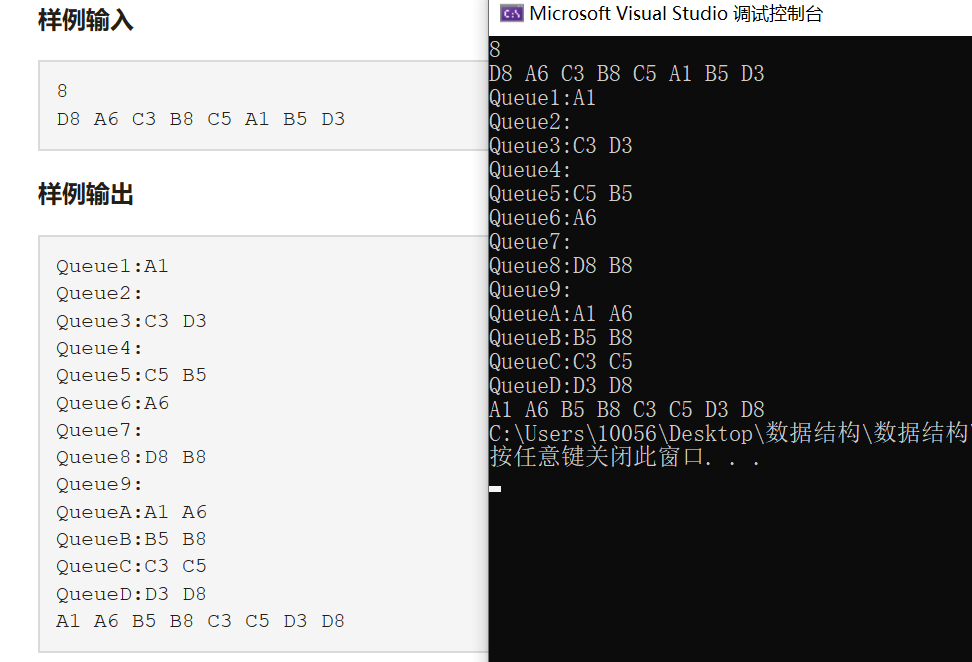# 扑克牌排序

1、poke结构体

typedef struct {
char a;
int b;
}poke;

2、数组型队列

queue<poke> color;
queue<poke> number;queue<poke> sorted;    

先建立9个队列，用于存放点数的大小，将卡牌依点数存放入各自的队列之中，然后再按队列1到队列9依次出队。

1. 输入n；
2. 循环n次；
2.1 输入p.a，输入p.b；
2.2 将p放入第p.b个数字队
3. i从1循环到9
3.1 循环到数字i队为空
3.1.1 p = number[i].front();
3.1.2 color[p.a - 'A' + 1].push(p);
3.1.3 number[i].pop();
4.i从1循环到4
4.1 循环到花色i队为空
4.1.1 p = color[i].front();
4.1.2 sorted.push(p);
4.1.3 color[i].pop();
5.输出sorted

1、时间复杂度

2、空间复杂度

3、相比冒泡排序

(1)时间复杂度：冒泡排序的最好时间复杂度为O(n)，最坏时间复杂度为O(n^2)，而该算法的时间复杂度为O(d*(n+r))。

(2)空间复杂度：冒泡排序为O(1)，基数排序为O(n+r)。

(3)冒泡排序每次只能移动两个元素，需要耗费的时间长，但是占用空间较小且容易理解，算法容易实现，适用于较小规模的排序；基数排序的效率高于其他比较性排序，适用于规模较大且关键字较小的情况。

#include<iostream>
#include<queue>

using namespace std;

typedef struct {
char a;
int b;
}poke;

int main()
{
queue<poke> color;
queue<poke> number;
poke p;
int n;
cin >> n;
for (int i = 0; i < n; i++) {
cin >> p.a >> p.b;
number[p.b].push(p);
}
for (int i = 1; i <= 9; i++) {
cout << "Queue" << i << ":";
while (!number[i].empty()) {
p = number[i].front();
cout << p.a << p.b << " ";
color[p.a - 'A' + 1].push(p);
number[i].pop();
}
cout << "\n";
}
queue<poke> sorted;
for (int i = 1; i <= 4; i++) {
cout << "Queue" << char('A' + i - 1) << ":";
while (!color[i].empty()) {
p = color[i].front();
cout << p.a << p.b << " ";
sorted.push(p);
color[i].pop();
}
cout << "\n";
}
while (!sorted.empty()) {
p = sorted.front();
cout << p.a << p.b << " ";
sorted.pop();
}
return 0;
}posted @ 2020-03-30 21:57  Tvivanomy(王林涛)  阅读(1005)  评论(2编辑  收藏  举报